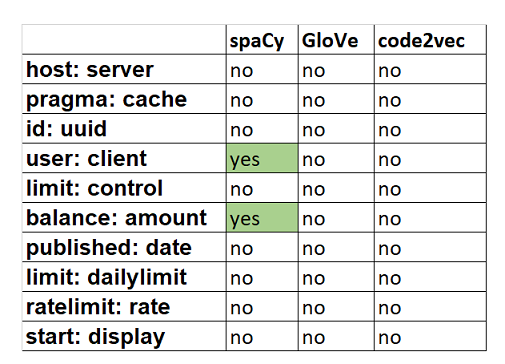# 用code2vec、glow和spaCy进行词嵌入

code2vec是一个神经模型，可以学习与源代码相关的类比。该模型是在Java代码数据库上训练的，但是你可以将其应用于任何代码。

1. 下载数据集

2. 下载词汇表

3. 提取字段名称

4. 标识化

5. 创建字段名称的数据集

6. 测试嵌入

7. 结论

### 2.下载词汇表

code2vec

1. 从code2vec GitHub页面下载模型，按照快速入门部分中的说明进行操作。

2. 使用gensim库加载。

model = word2vec.load_word2vec_format(vectors_text_path, binary=False)

GloVe

1. 从网站下载一个GloVe词汇表。我们选了最大的一个，因为这样它就更有可能找到我们所有的单词。你可以选择下载它的位置，但为了方便起见，最好将其存储在工作目录中。

2. 手动加载GloVe词汇表。

embeddings_dict = {}
with open("../glove/glove.6B.300d.txt", 'r', encoding="utf-8") as f:
for line in f:
values = line.split()
word = values
vector = np.asarray(values[1:], "float32")
embeddings_dict[word] = vector

spaCy

nlp = spacy.load(‘en_core_web_lg’).

### 3.提取字段名

OpenAPI规范名称的整个列表可以从scripts/fetch-list.sh文件或使用以下函数（对于Windows）获取：

def getListOfFiles(dirName):
listOfFile = os.listdir(dirName)
allFiles = list()
for entry in listOfFile:
fullPath = posixpath.join(dirName, entry)
if posixpath.isdir(fullPath):
allFiles = allFiles + getListOfFiles(fullPath)
else:
allFiles.append(fullPath)

return allFiles

def get_fields_from_schema(o: Schema) -> Sequence[str]:
return [
*(o['properties'].keys() if ('properties' in o) and (type(o['properties']) == type({})) else []),
*(sum([
get_fields_from_schema(schema) for schema in o['properties'].values() if not ('$ref' in schema) and type(schema) == type({})], []) if ('properties' in o) and ($        *(get_fields_from_schema(o['additionalProperties']) if ('additionalProperties' in o) and (type(o['additionalProperties']) == type({})) else []),
*(get_fields_from_schema(o['items']) if ('items' in o) and  (type(o['items'] == type({}))) else []),
]

def get_fields_from_schemas(o: Mapping[str, Union[Schema, Reference]]) -> Sequence[str]:
return sum([get_fields_from_schema(cast(Schema, maybe_schema)) for maybe_schema in o.values() if not ('\$ref' in maybe_schema) and (type(maybe_schema) == type({}))], [])

def get_fields_from_components(o: Components) -> Sequence[str]:
return [
*(get_fields_from_schemas(o['schemas']) if 'schemas' in o else []),
]

def get_fields(o: OpenAPIObject) -> Sequence[str]:
return [
*(get_fields_from_components(o['components']) if 'components' in o else []),
]

### 4.标识化

def camel_case(example):
if  any(x in example for x  in string.punctuation)==True:
return False
else:
if any(list(map(str.isupper, example[1:-1])))==True:
return True
else:
return False

def camel_case_split(word):
idx = list(map(str.isupper, word))
case_change = 
for (i, (x, y)) in enumerate(zip(idx, idx[1:])):
if x and not y:
case_change.append(i)
elif not x and y:
case_change.append(i+1)
case_change.append(len(word))
return [word[x:y] for x, y in zip(case_change, case_change[1:]) if x < y]

def tokenizer(mylist):
tokenized_list=[]
for word in mylist:

if '_'  in word:
splitted_word=word.split('_')
for elem in splitted_word:
if camel_case(elem):
elem=camel_case_split(elem)
for el1 in elem:
tokenized_list.append(el1.lower())
else:
tokenized_list.append(elem.lower())
elif '-' in word:
hyp_word=word.split('-')
for i in hyp_word:
if camel_case(i):
i=camel_case_split(i)
for el2 in i:
tokenized_list.append(el2.lower())
else:
tokenized_list.append(i.lower())
elif camel_case(word):
word=camel_case_split(word)
for el in word:
tokenized_list.append(el.lower())
else:
tokenized_list.append(word.lower())
return(tokenized_list)
tokenizer(my_word)

### 5.创建字段名的数据集

def dict_dataset(datasets):
dataset_dict={}
for i in datasets:
with open(i, 'r') as foo:
if col:
mylist = list(dict.fromkeys(col))
tokenized_list=tokenizer(mylist)
dataset_dict.update({i: tokenized_list})
else:
continue
return (dataset_dict)

### 6.测试嵌入

code2vec和GloVe

not_identified_c2v=[]
count_not_indent=[]
total_number=[]

for ds in test1:
count=0
for i in data[ds]:
if not i in model:
not_identified_c2v.append(i)
count+=1
count_not_indent.append(count)
total_number.append(len(data[ds]))

total_code2vec=sum(count_not_indent)/sum(total_number)*100

spaCy

spaCy词汇表不同，因此我们需要相应地修改代码：

not_identified_sp=[]
count_not_indent=[]
total_number=[]

for ds in test1:
count=0
for i in data[ds]:
f not i in nlp.vocab:
count+=1
not_identified_sp.append(i)
count_not_indent.append(count)
total_number.append(len(data[ds]))

total_spacy=sum(count_not_indent)/sum(total_number)*100

test_dictionary={'host': 'server',
'pragma': 'cache',
'id': 'uuid',
'user': 'client',
'limit': 'control',
'balance': 'amount',
'published': 'date',
'limit': 'dailylimit',
'ratelimit': 'rate',
'start': 'display',
'data': 'categories'}

from scipy.spatial import distance

for k, v in test_dictionary.items():
input_word = k
p = np.array([nlp.vocab[input_word].vector])
closest_index = distance.cdist(p, vectors).argsort()[::-1][-100:]
word_id = [ids[closest_ind] for closest_ind in closest_index]
output_word = [nlp.vocab[i].text for i in word_id]
#output_word
list1=[j.lower() for j in output_word]
mylist = list(dict.fromkeys(list1))[:50]
count=0
if test_dictionary[k] in mylist:
count+=1
print(k,count, 'yes')
else:
print(k, 'no')### 结论

spaCy比其他算法更快。spaCy词汇表的读取速度比glow或code2vec词汇表快5倍。然而，在使用该算法时，缺少内置函数（如similar_by_vector和similar_word）是一个障碍。

http://panchuang.net/

sklearn机器学习中文官方文档：
http://sklearn123.com/

http://docs.panchuang.net/